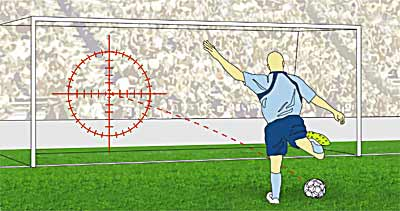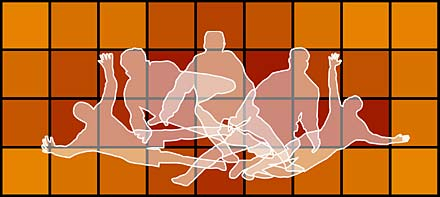By John Haigh

## Penalty tactics in soccer

When taking a penalty kick, a player ought to score (provided the referee applies all the rules). He can fail in two ways: by missing the target altogether, or having a shot on target stopped by the goalkeeper. We can look on taking a penalty as a game, in which both the shooter, S, and the goalkeeper, G, must choose from a finite list of tactics. For each choice made (S might aim low right, G might dive to the left), we can estimate a payoff - the chance a goal will result. The shooter's aim is to maximise his expected payoff, G aims to minimise the same quantity. This is the classical set-up of a zero-sum game.### Two tactics for each

To illustrate the possibilities, suppose that each player has just two choices: S may aim to the left or to the right of the goal, G may dive to his left or right. (So if S aims left, and G dives right, ball and goalkeeper should be close together.) If the shot is on target, and G dives the wrong way, a goal is inevitable; if G dives the right way, suppose he has a 50% chance of stopping a
shot on target. Finally, take the player to be more accurate when shooting to the right - he is then on target 90% of the time, but only 70% of the time when shooting left. The payoff matrix, which gives S's percentage chance of scoring, is then

G dives left G dives right
S aims left 70 35
S aims right 45 90

We assume that both players are fully aware of these figures. There is a standard method of finding the optimal tactics for both in this situation. Each should randomise, i.e. choose one of their tactics with a certain probability. And each should choose those probabilities that make his average payoff the same, whatever the other does. So let p be the chance that S shoots left;
if G dives left (the first column of the table), the average payoff for S is

70p+45(1-p)=45+25p.

If G dives right (the second column), then his average payoff is

35p+90(1-p)=90-55p.

The equation

45+25p=90-55p

solves to give

p=45/80=56.25%.

Thus S should aim left with probability 56.25%, or right with the remaining probability 43.75%; and his chance of scoring, the corresponding average payoff, is just over 59%. He uses a mixed strategy.

It may seem odd that S is advised to have a bias towards shooting left here, as his chance of hitting the target is lower. But the explanation arises when we use similar methods to find the best tactics for G: G should go to his left with probability 55/80=68.75%, and to his right with probability 31.25%. So when S aims left, G's best strategy sends him towards the ball only just over 30% of
the time, which is low enough to more than compensate for the extra chance that S will miss the target. Indeed, if S were to use the naive strategy of always shooting to the right, where he is more accurate, and G could read his mind, the chance of scoring would drop from 59% to 45%.Whenever S and G have a choice of only two tactics, finding their best strategy for a given payoff matrix is quite straightforward. First we should check that it will be sensible for both players to consider using both tactics. That would not be the case, for instance, if both entries on the top row had been at least as high as the corresponding entries on the bottom row, because then,
whatever G did, S would have been at least as well off aiming left as right - one of his choices dominates the other. If either player has one tactic that dominates the other, we can eliminate the dominated tactic: and then it will be perfectly obvious what both players should do.

When there are no dominating tactics, the procedure described in the example will always lead to the optimal (mixed) strategy for the two players, and hence the chance a goal is scored. If both players use optimal tactics, it does not matter if they spell out their mixed strategy to their opponent. The goalkeeper could write his probabilities on the crossbar, the shooter could tattoo
his intentions on his boots; the chance of scoring is not affected. But departure from these optimal intentions can always be exploited by the opponent. For example, suppose S decides to give himself an 80% chance of aiming left, and a 20% chance of aiming right. Then if G dives left, the average payoff is $0.80\times0.70+0.20\times0.45=0.65$, while if he dives right, the average payoff is $0.80\times0.35+0.20\times0.90=0.46$. Since G wishes the payoff to be as small as possible, he should now always dive right, and the scoring chance has dropped from the optimal 59% down to 46%. World Cups can be won or lost on such differences.

### More than two tacticsMissed...

In reality, both players have a choice of more than two tactics. The shooter might aim low or high; left, right or centre; and go for either accuracy or power - that would already give twelve choices. But the main ideas from the example above carry through. Some strategies may dominate others, and so the size of the payoff matrix can be reduced; the optimal strategy for both players will, in general, be mixed - they choose from their alternatives according to a probability distribution; and departure from an optimal strategy allows the other to do better. The main change is that the procedure for finding the optimal strategies can be much more complicated. An algorithm known as the simplex method can be used to find these optimal strategies, but it will not be pursued here.
Instead, we will look at three examples, which illustrate different types of solution, even when both players have three tactics only. Use A, B and C to denote the tactics for the shooter S, and I, II and III for G.

G I G II G III
S A 45 50 35
S B 50 40 45
S C 60 30 65

At first sight, there seem to be no dominated tactics, but if we look a bit harder, we can find some! Compare the average of the top row and the bottom row with the entries on the middle row, one at a time. The three averages are 52.5, 40 and 50 which does dominate the middle row. That means that if the shooter uses a 50-50 mix of A and C, his scoring chance is always at least as
high as when he uses B, and sometimes higher. So we can strike out row B - it will never be optimal for S to use that tactic. Now, on the reduced matrix, compare the first column with the average of the other two: it is higher in both components, so since the goalkeeper wants the payoff to be as small as possible, he is better off using a 50-50 mixture of II and III, than using I. We also strike
out the first column, leaving the payoff matrix as

G II G III
S A 50 35
S C 30 65

There are no more dominated strategies, and the procedure for a two-by-two matrix leads to the optimal strategies: the shooter should use A 70% of the time, otherwise C; and G should use II with probability 60%, otherwise III. The chance of a goal is then 44% (all of which you should check).

For another example, suppose the percentage chances of scoring are

G I G II G III
S A 60 50 80
S B 80 50 50
S C 60 80 20

Here there are no obvious dominated strategies, and if we follow the hint that the shooter should use a mixed strategy that gives the same payoff, whatever the goalkeeper does, suppose the shooter uses his three tactics with respective probabilities x, y and 1-x-y. Looking at each column in turn, the three mean payoffs are

• 60x + 80y + 60(1-x - y) = 60 + 20y,
• 50x + 50y + 80(1-x - y) = 80 - 30x - 30y
• 80x + 50y + 20(1-x - y) = 20 + 60x + 30y.

Equating these quantities leads to y=0 and x=2/3, which means that the shooter should never use B, and he should be twice as likely to use A as C. His scoring chance is then 60%. For the goalkeeper, the corresponding equations lead to the conclusion that he should use I, II or III with respective chances 1/3, 4/9 and 2/9.

But this is not the complete story: although the shooter has a unique optimal strategy, the goalkeeper has another one, quite different. He could use II and III only, with respective probabilities 2/3 and 1/3. You can check that the mean payoffs, for the three choices for the shooter, are then 60, 50 and 60; but since the shooter should know never to use B, this apparent reduction in the
scoring chance is illusory! In fact, the goalkeeper has infinitely many optimal strategies, as he could mix the two we have found in any proportions he likes.

As a final example, suppose the payoff matrix is

G I G II G III
S A 70 60 30
S B 80 40 85
S C 40 80 90

These might be realistic numbers when the shooter's choices correspond to aiming left, middle or right, while the goalkeeper opts to dive left, stay still, or dive right. This time, both opponents have a unique best strategy that gives a positive probability to each of their choices. The shooter should use the three choices in proportions 4:2:3, while the goalkeeper's probabilities should be
in the ratios 46:45:8 (leading to fractions expressed as 9ths, or 99ths, respectively). The chance of a goal is 560/9, just over 62%.Which way?

In all these examples, we can ask whether the solution we have found looks sensible (a good precaution after a calculation in a mathematical model). In the first example, we were able to see why the counter-intuitive solution - shoot in the direction where your accuracy is less - was still sensible. We might also have noted that the overall chance of scoring must be within the range of figures
in the payoff matrix: and, in that same example, 59% is near the middle of the two extreme values of 35% and 90%. In this final example, the algebra says that the goalkeeper should hardly ever dive right; and notice that the two highest entries, 85 and 90 (both bad for G), are in the column where G would dive right.

It should be easy enough for you to set up plausible payoff matrices, either for your own soccer games, or for contests between your favourite professional players. To prevent the arithmetic getting too complex, stick to at most three choices for each player. And seek to assure yourself that your answers, describing optimal tactics for both players and the chance of a goal, make sense. (Be warned that the method described here is not guaranteed to work for every possible set of payoffs you could think of - sometimes the equations will lead to apparently negative probabilities! You would then need to know about the simplex method, mentioned earlier.)